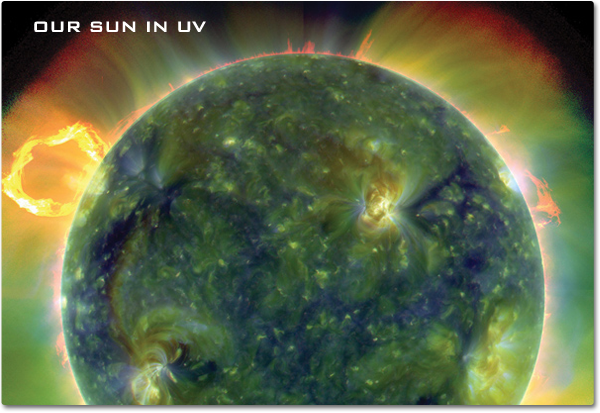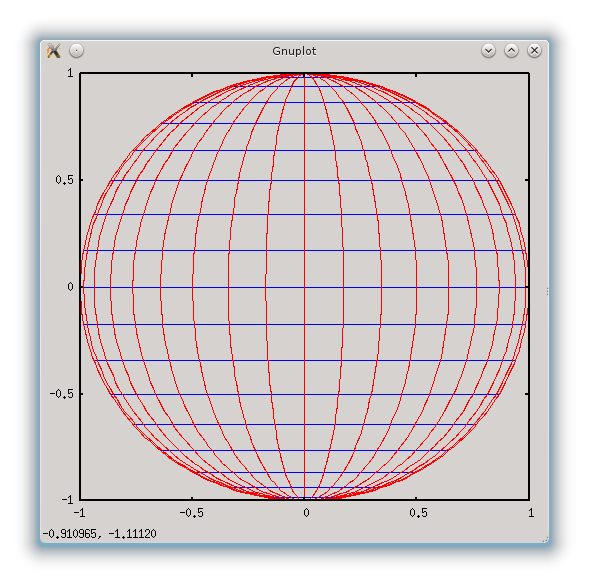## Solar co-ordinate fun

Fri 24 April 2015

Transforming co-ordinate systems between pixel images and the surface of a sphere is probably not most peoples' idea of fun, but I enjoy it. To sugar the pill take a look at this fabulous image:The Sun in ultraviolet taken by the Solar Dynamics Observatory. Credit: NASA/SDO/AIA

If you see an interesting feature on the surface of the Sun, you can specify its position by its pixel co-ordinates. The image above is 600 pixels across by 413 pixels high, so I can say that the interesting, yellow feature (a cluster of magnetic field loops in an active region) to the right of the image's centre is located at (380,210), that is, 380 pixels in from the left side, and 210 down from the top edge of the image.

This is a bit unsatisfactory, so it might be better to use a different co-ordinate system to say the feature is centred on the point (80,90) measured relevant to the centre of the Sun's disc, i.e. 80 pixels to the right and 90 pixels up from the centre.

But this isn't very useful because the Sun is a sphere, not a flat disc. We use longitude and latitude to specify our location on a spherical Earth, so we can use something similar for the Sun. Let's say heliographic latitude is zero along a line drawn horizontally through the centre of the Sun; this is the solar equator. The North pole is +90°N and will be at the top and -90°S at the bottom will be the South pole. Of course, this assumes the Sun has been correctly orientated in the image.

We can define heliographic longitude so that 0° lies on a vertical line that runs through the two poles. This is sometimes called the solar meridian. A point with a longitude of 90° lies on the right edge of the Sun (referred to as the west limb by solar observers) and a point with a longitude of -90° lies on the left edge (east limb).

Unlike the longitude and latitude system of the Earth which rotates with the Earth, this solar co-ordinate system is fixed to our viewpoint. This means that the Sun's rotation will cause features to move from left to right, e.g. the longitude of a sunspot will increase over time, though its latitude won't be affected. The Sun is not a solid body: it takes about 25 days to rotate once at the equator, and somewhat longer to complete a rotation nearer the poles.

The image below illustrates what lines of constant longitude and latitude look like on a 2D image. The central vertical red line is the solar meridian at longitude 0°, and the first red curve to its right is 10°, and the next is 20°, and so on. The solar equator is the central horizontal blue line, and the line of 10°N latitude is immediately above it.Solar lines of longitude (red) and latitude (blue) in intervals of 10°. The plot was generated using the venerable plotting program gnuplot using this script.

Points of equal latitude (blue) form horizontal straight lines, but points of longitude (red) form curves. For example, the longitude line for -80° is a curve very close to the left edge of the Sun. This explains why features near the limb of the Sun will appear squashed, which is referred to as foreshortening by astronomers.

## Some mathematics

If you don't like equations then you won't like this bit. Sorry.

Consider a point on the solar surface that's at longitude $$\phi$$ and latitude $$\theta$$. Our first aim is to calculate the pixel co-ordinates of this point. Let's take the point (0,0) to be at the centre of the Sun's disc (not the image, which may be different). A bit of spherical trigonometry and 3D to 2D projection (which I'll not get into here) yields these equations:

\begin{eqnarray} x&=& R\cos\theta\sin\phi \label{x} \\\ y&=& R\sin\theta \label{y} \end{eqnarray}

where $$R$$ is the radius of the Sun in pixels, i.e. half the diameter.

We can now turn these equations around to work in the opposite direction - given $$(x,y)$$ pixel co-ordinates, calculate the longitude $$\phi$$ and latitude $$\theta$$. Let's take (\ref{y}) first which tells us that $$\theta$$ is:

$$\theta = \sin^{-1}\frac{y}{R}$$

The -1 indicates we need to perform the sin function in reverse, sometimes called arcsin or inverse sin. This can be done by using the shift or inv button on a scientific calculator. The angle obtained needs to be between -90° and +90° where minus means South (or below centre).

Now we have $$\theta$$ we can use it to calculate the latitude $$\phi$$ by re-arranging (\ref{x}):

$$\phi = \sin^{-1}\frac{x}{R\cos\theta}$$

and again $$\phi$$ should be between -90° and +90° where minus means east (or left of centre).

You can also use spreadsheets to perform these calculations, though remember that spreadsheet trig functions (sin, cos etc) usually work in radians rather than degrees. This spreadsheet shows an example of calculations in either direction: download ods or download xls.